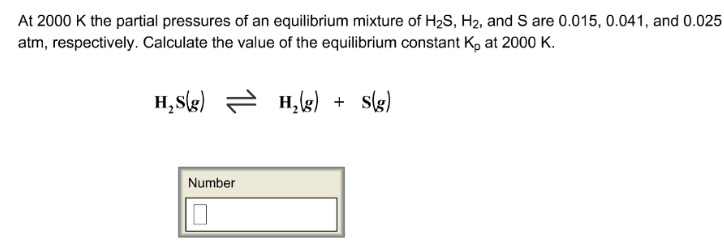# Problem: At 2000 K the partial pressures of an equilibrium mixture of H2S, H2, and S are 0.015, 0.041, and 0.025 atm, respectively. Calculate the value of the equilibrium constant Kp at 2000 K. H2S (g) ⇌ H2 (g) + S (g)

###### FREE Expert Solution
85% (6 ratings)###### Problem Details

At 2000 K the partial pressures of an equilibrium mixture of H2S, H2, and S are 0.015, 0.041, and 0.025 atm, respectively. Calculate the value of the equilibrium constant Kp at 2000 K.

H2S (g) ⇌ H(g) + S (g)